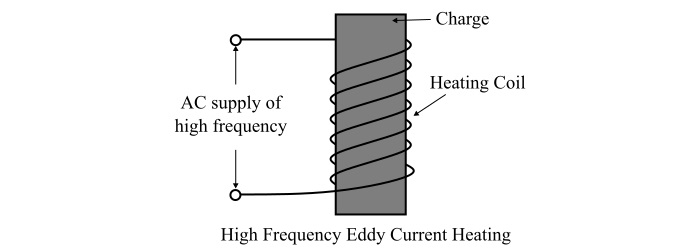## Eddy Current Heating

When the heating of the charge is done by the induced eddy currents in charge, it is known as eddy current heating.

To heat the charge by eddy current heating process, the charge is placed inside a current carrying coil. The current is the high frequency alternating current. For this reason, this method is also called the high frequency eddy current heating. The alternating magnetic field produced by the coil sets up eddy currents in the charge to be heated. Consequently, the charge gets heated-up. The coil used for creating the magnetic field is known as heater coil or work coil.

In this method of electric heating, it is the eddy current loss which is mainly responsible for the production of heat. Although, the hysteresis loss also contributes to some extent, when the charge to be heated is made of magnetic material. The figure shows the simplified schematic of high frequency eddy current heating.As the power loss due to eddy currents (i.e., eddy current loss) is given by,

$$\mathrm{\mathit{P_{e}}\propto \mathit{B}^{\mathrm{2}}\mathit{f}^{\mathrm{2}}}$$

Where, B is the magnetic flux density and f is the supply frequency.

Therefore, the heat produced in the eddy current heating can be controlled by controlling the flux density (B) and the supply frequency (f). The eddy current loss is greatest on the surface and decreases when we go deep inside the charge to be heated. The depth of the penetration of the eddy currents into the charge is given by,

$$\mathrm{\mathit{d}\:=\:\frac{1}{2\pi}\sqrt{\frac{\rho \times 10^{\mathrm{9}}}{\mu _{\mathit{r}}\times \mathit{f}}}\:\mathit{cm}}$$

Where,

• ρ is the resistivity of molten metal.

• f is the supply frequency.

• $\mu _{\mathit{r}}$ is the relative permeability of the material of the charge.

$$\mathrm{\because \mathit{d}\propto \frac{1}{\sqrt{\mathit{f}}}}$$

Therefore, by changing the supply frequency, the eddy current heating can be restricted to any desired depth of the charge to be heated. The supply frequency usually used for the eddy current heating ranges from 10 kHz to 40 kHz.

## Advantages of Eddy Current Heating

The following are the advantages of the eddy current heating −

• With the eddy current heating, the temperature control is very easy.

• As the heat is produced in the body to be heated up directly. Therefore, the wastage of heat is very less.

• The heating coils are not required to fit closely around the object being treated.

• The heat penetration into the metal surface can be controlled to any desired depth.

• The eddy current heating method is quick, clean and convenient.

• Unskilled personal can also operate the heating equipment easily.

• The eddy current heating can easily take place in vacuum or any other special atmosphere.

• By using suitable timing devices, the amount of heat produced by the eddy current heating method can be accurately controlled.

## Disadvantages of Eddy Current Heating

The disadvantages of the high frequency eddy current heating are given as follows −

• In case of eddy current heating, the cost of heat production is high.

• The initial cost of the equipment used for eddy current heating is high.

• The efficiency of the equipment used for eddy current heating is very low, about less than 50%.

## Applications of Eddy Current Heating

Some of the applications of eddy current heating are given as follows −

• The eddy current heating is used for surface hardening process.

• The eddy current heating is a convenient method for annealing.

• Eddy current heating also used for soldering precisely.

• It is used for drying of paints.

• Eddy current heating is also employed for welding process.

• It is used for forging of bolt heads and rivet heads.

• It is also used for melting of various metals.

• For sterilization of surgical instruments, the eddy current heating is used.